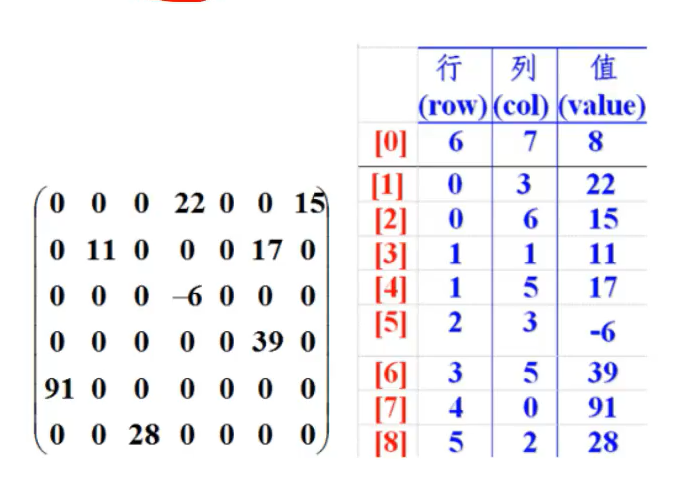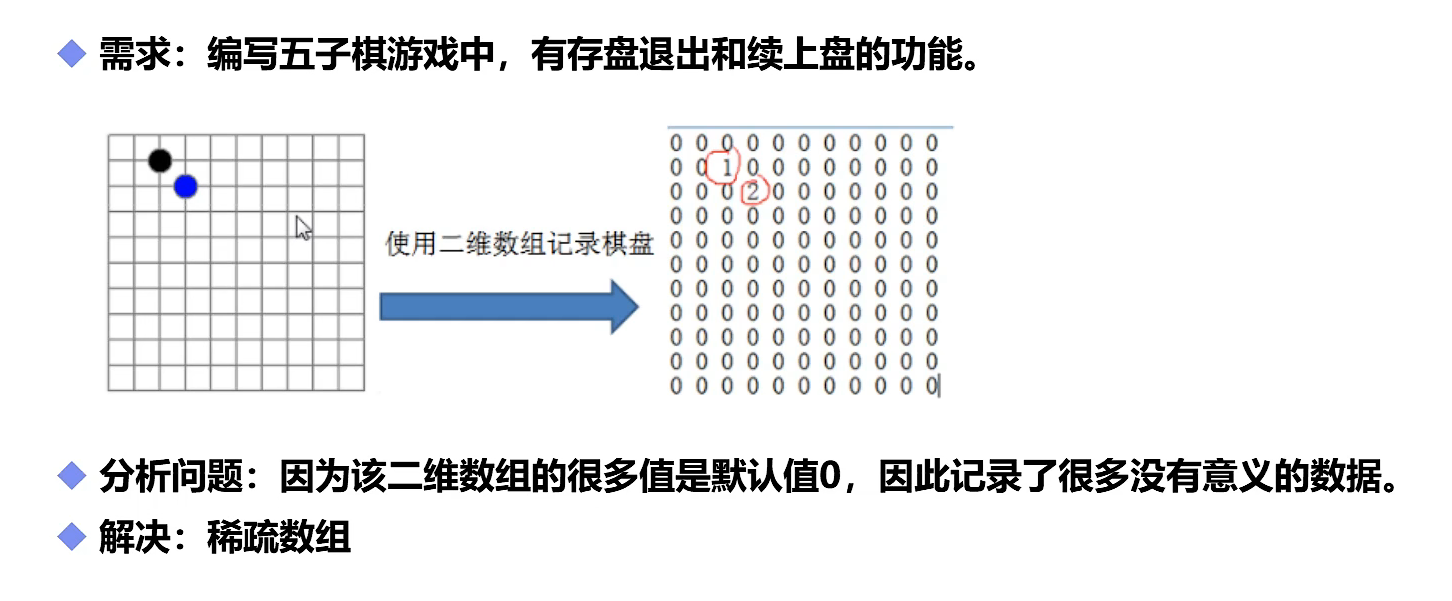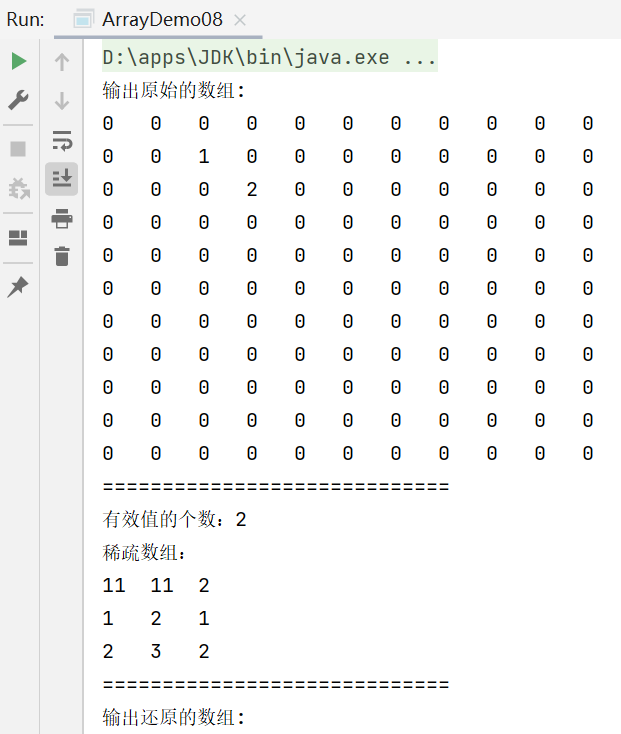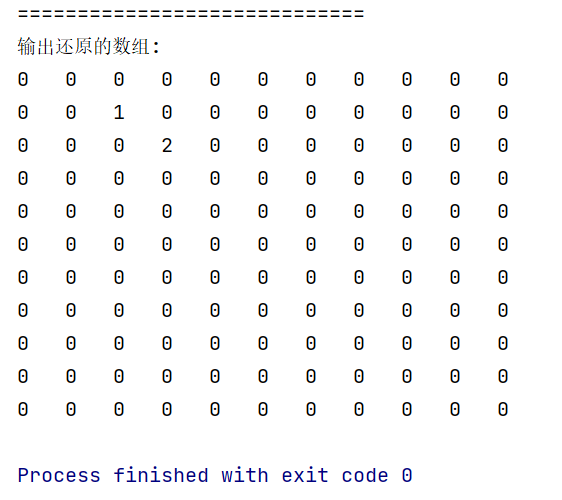# Java数组

## 9.稀疏数组

• 当一个数组中大部分元素为0，或者为同一值的数组时，可以使用稀疏数组来保存该数组。

• 稀疏数组的处理方式是：

• 记录数组一共有几行几列，有多少个不同的值

• 把具有不同值 的元素和行列及值记录在一个小规模的数组中，从而缩小程序的规模``````package li.bolog.array;
​
//创建稀疏数组
public class ArrayDemo08 {
public static void main(String[] args) {
​
//1.创建一个二维数组 11*11  0：代表没有棋子    1：黑棋    2：白棋
int[][] array1=new int;
array1=1;
array1=2;
​
//输出原始的数组
System.out.println("输出原始的数组:");
for (int[] ints:array1) {
for (int anInt:ints){
System.out.print(anInt+"\t");
}
System.out.println();
}
System.out.println("=============================");
​
//转换为稀疏数组保存
//获取有效值的个数sum
int sum=0;
for (int i = 0; i < 11; i++) {
for (int j = 0; j < 11; j++) {
if (array1[i][j] != 0) {
sum++;
}
}
}
System.out.println("有效值的个数："+sum);
​
//2.创建一个稀疏数组
/*样式如图所示
一共有sum个有效值，则行数是sum+1，记录总行、列、有效值个数占一行，记录有效值占sum行
3列则是固定的，分别是行、列、值
*/
int[][] array2=new int[sum+1];
​
array2=11;
array2=11;
array2=sum;
​
//遍历二维数组，将非零的值存放到稀疏数组中
int count=0;
for (int i = 0; i < array1.length; i++) {//array1.length代表array1的行数
for (int j = 0; j < array1[i].length; j++) {//array1[i].length代表列数
if (array1[i][j] != 0) {
count++;//count数值从一开始，因为第0行已经存了稀疏数组的总行、列、有效值个数
array2[count]=i;//从当前行的第一个值存有效值的行数
array2[count]=j;//从当前行的第二个值存有效值的列数
array2[count]=array1[i][j];//从当前行的第三个值存放有效值数值
}
}
​
}
//输出稀疏数组
​
System.out.println("稀疏数组：");
​
for (int i = 0; i < array2.length; i++) {
System.out.println(array2[i]+"\t"
+array2[i] +"\t"
+array2[i] +"\t");
​
}
​
System.out.println("=============================");
​
//稀疏数组还原
//1.读取稀疏数组
//array2代表原来数组总行数   array2代表原来数组总列数
int[][] array3 = new int[array2][array2];
​
/*
稀疏数组：
11  11  2
1   2   1
2   3   2
*/
//2.给其中元素还原值
for (int i = 1; i < array2.length; i++) {//array.length为稀疏矩阵的行数，这里具体值为3
array3[ array2[i] ][ array2[i] ] = array2[i];//这里只填入了有效值，其他的0值在array3创建时已经隐式初始化
}
​
//3.打印array3
System.out.println("输出还原的数组:");
for (int[] ints:array3) {
for (int anInt:ints){
System.out.print(anInt+"\t");
}
System.out.println();
}
​
}
}``````